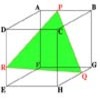#### You may also like### Cut Cube

Find the shape and symmetries of the two pieces of this cut cube.### Cushion Ball

The shortest path between any two points on a snooker table is the straight line between them but what if the ball must bounce off one wall, or 2 walls, or 3 walls?### Plane to See

P is the midpoint of an edge of a cube and Q divides another edge in the ratio 1 to 4. Find the ratio of the volumes of the two pieces of the cube cut by a plane through PQ and a vertex.

# Napoleon's Hat

##### Age 16 to 18 Challenge Level:

Why do this problem?
Learners can investigate using the interactivity, make a conjecture and then try to prove their own conjecture.

It can be an exercise in coordinates.

It is useful for learners to appreciate that the symmetry of the result makes it unecessaryto work out all three distances.

Possible approach
Ideally learners would work independently on this problem.

Key question
How do we find the coordinates of the centroid of an equilateral triangle?

Possible support
GeoGebra is FREE educational mathematics software and your learners can experiment with it and draw their own dynamic diagrams.